Function Repository Resource:

# PowerTotal

Compute the total of a list of numbers all taken to some power

Contributed by: Seth J. Chandler
 ResourceFunction["PowerTotal"][y,n,x] takes x to the yth power and then applies the Total function using level specification n. ResourceFunction["PowerTotal"][y,n] represents an operator form of ResourceFunction["PowerTotal"] that can be applied to an expression x. ResourceFunction["PowerTotal"][y] represents an operator form of ResourceFunction["PowerTotal"] that, when applied to x, computes the total at the top level. ResourceFunction["PowerTotal"][] represents an operator form of ResourceFunction["PowerTotal"] that, when applied to x, takes the sum of the squares.

## Details and Options

Both x and y can be real, complex or symbolic.
The level specification n may take the form of:
an integer n, meaning the summation is performed at all levels down to level n;
an integer n wrapped with List, meaning the summation is performed at level n only; and
a List of two integers {n1,n2}, meaning the summation is performed at all levels n1 through n2.
See the documentation for Total for a more complete explanation of how the level specification works there.

## Examples

### Basic Examples (4)

Compute the sum of the squares of a list:

 In:=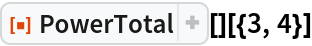Out=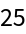Compute the sum of the cubes of a list with symbolic parts:

 In:=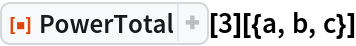Out=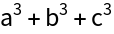Compute the sum of the cubes of a symbolic array:

 In:=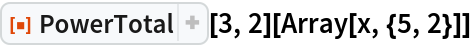Out=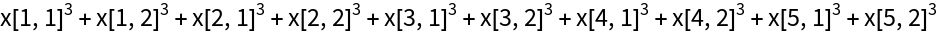Create an operator which when confronted with an expression computes the sum of its square roots:

 In:=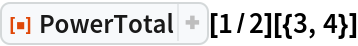Out=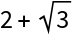### Scope (5)

The power may be complex, as may the list:

 In:=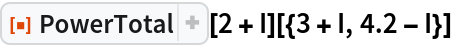Out=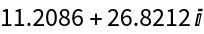The level specification can affect the results when the data has more than one dimension:

 In:=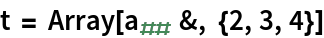Out=The default behavior is to apply at level 1:

 In:=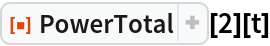Out=Apply the total down to level 2:

 In:=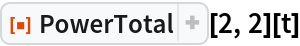Out=Apply the total in the last two dimensions:

 In:=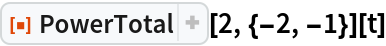Out=### Applications (3)

Use PowerTotal to conduct ordinary least squares linear regression by finding the values of two parameters a and b that minimize the sum of the squared distances between the actual value of the independent variable and a value that depends on a and b:

 In:=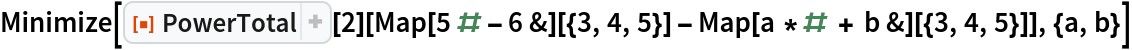Out=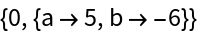Use PowerTotal to perform "Tikhonov" (ridge) regression:

 In:=Out=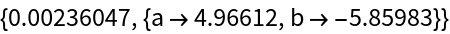Use PowerTotal to perform "LASSO" regression:

 In:=Out=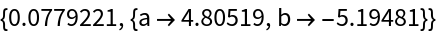### Properties and Relations (2)

PowerTotal[] is the same as the square of the results from the Norm function if the arguments it confronts are real-valued, but is not necessarily the same if the values it confronts are complex:

 In:=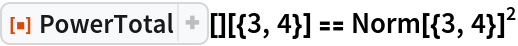Out=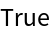In:=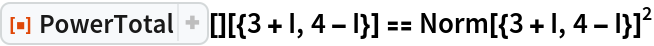Out=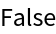PowerTotal is the same as Total if the power argument is 1:

 In:=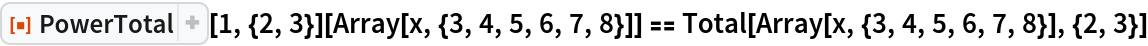Out=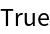Seth J. Chandler

## Version History

• 1.0.0 – 30 December 2019